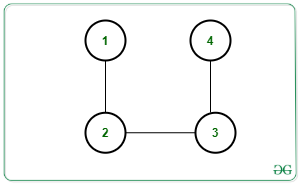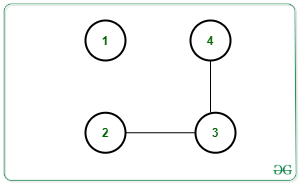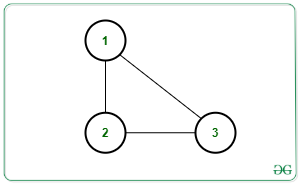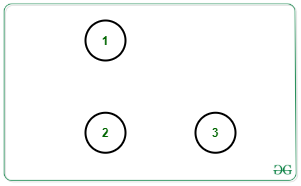Open in App
Not now

# Maximum number of edges to be removed to contain exactly K connected components in the Graph

• Difficulty Level : Hard
• Last Updated : 27 Aug, 2021

Given an undirected graph G with N nodes, M edges, and an integer K, the task is to find the maximum count of edges that can be removed such that there remains exactly K connected components after the removal of edges. If the graph cannot contain K connect components, print -1.

Examples:

Input: N = 4, M = 3, K = 2, Edges[][] = {{1, 2}, {2, 3}, {3, 4}}Output: 1
Explanation:
One possible way is to remove edge [1, 2]. Then there will be 2 connect components as shown below:Input: N = 3, M = 3, K = 3, Edges[][] = {{1, 2}, {2, 3}, {3, 1}}Output: 3
Explanation: All edges can be removed to make 3 connected components as shown below:Approach: To solve the given problem, count the number of connected components present in the given graph. Let the count be C. Observe that if C is greater than K then no possible edge removal can generate K connected components as the number of connected components will only increase. Otherwise, the answer will always exist.

Following observations need to be made in order to solve the problem:

• Suppose C1, C2, …, Cc, are the number of node in each connected component. Then, each component must have edges as C1 – 1, C2 – 1, …, C-1 after edges are removed. Therefore,

C1 – 1 + C2 – 1 + … + Cc – 1 = C1 + C2 + … + Cc – C = N – C, where N is the number of nodes.

• The above condition will give us the C connected components by removing M – (N – C) edges as N – C edges are needed to make C components. To get K components, (K – C) more edges must be removed.
• Hence, the total count of edges to be removed is given by:

M – (N – C) + (K – C) = M – N + K

Follow the steps below to solve the problem:

1. Count the number of connected components present in the given graph. Let the count be C.
2. If C is greater than K, print -1.
3. Else print M – N + K where N is the number f nodes, M is the number of edges and K is the required number of connected components.

Below is the implementation of the above approach:

## C++

 `// C++ program for the above approach` `#include ` `using` `namespace` `std;`   `class` `Graph ` `{` `  ``public``:` `    ``int` `V;` `    ``map<``int``, vector<``int``>> adj;`   `    ``Graph(``int``);` `    ``void` `addEdge(``int``, ``int``);` `    ``void` `DFS(``int``, vector<``bool``> &);` `} * g;`   `// Constructor` `Graph::Graph(``int` `V) ` `{` `  `  `  ``// No. of vertices` `  ``this``->V = V;` `  `  `  ``// Dictionary of lists` `  ``for``(``int` `i = 1; i <= V; i++) ` `    ``adj[i] = vector<``int``>();` `}`   `// Function to add edge` `// in the graph` `void` `Graph::addEdge(``int` `v, ``int` `w)` `{` `  ``adj[v].push_back(w);` `  ``adj[w].push_back(v);` `}`   `// Function to perform DFS` `void` `Graph::DFS(``int` `s, vector<``bool``> &visited) ` `{` `  `  `  ``// Create a stack for DFS` `  ``stack<``int``> stack;`   `  ``// Push the current source node` `  ``stack.push(s);` `  ``while` `(!stack.empty()) ` `  ``{` `    `  `    ``// Pop a vertex from stack` `    ``// and print it` `    ``s = stack.top();` `    ``stack.pop();`   `    ``// Traverse adjacent vertices` `    ``// of the popped vertex s` `    ``for``(``auto` `node : adj[s])` `    ``{` `      ``if` `(!visited[node]) ` `      ``{` `        `  `        ``// If adjacent is unvisited,` `        ``// push it to the stack` `        ``visited[node] = ``true``;` `        ``stack.push(node);` `      ``}` `    ``}` `  ``}` `}`   `// Function to return the count` `// edges removed` `void` `countRemovedEdges(``int` `N, ``int` `M, ``int` `K)` `{` `  ``int` `C = 0;`   `  ``// Initially mark all vertices` `  ``// as not visited` `  ``vector<``bool``> visited(g->V + 1, ``false``);`   `  ``for``(``int` `node = 1; node <= N; node++) ` `  ``{` `    `  `    ``// If node is unvisited` `    ``if` `(!visited[node])` `    ``{` `      `  `      ``// Increment Connected` `      ``// component count by 1` `      ``C = C + 1;`   `      ``// Perform DFS Traversal` `      ``g->DFS(node, visited);`   `      ``// Print the result` `      ``if` `(C <= K)` `        ``cout << M - N + K << endl;` `      ``else` `        ``cout << -1 << endl;` `    ``}` `  ``}` `}`   `// Driver Code` `int` `main(``int` `argc, ``char` `const` `*argv[]) ` `{` `  ``int` `N = 4, M = 3, K = 2;`   `  ``// Create Graph` `  ``g = ``new` `Graph(N);`   `  ``// Given Edges` `  ``g->addEdge(1, 2);` `  ``g->addEdge(2, 3);` `  ``g->addEdge(3, 4);`   `  ``// Function Call` `  ``countRemovedEdges(N, M, K);` `}`   `// This code is contributed by sanjeev2552`

## Java

 `// Java program to implement  ` `// the above approach ` `import` `java.util.*;` `class` `GFG` `{`   `  ``static` `ArrayList> graph;`   `  ``// Function to perform DFS` `  ``static` `void` `DFS(``int` `s, ``boolean``[] visited) ` `  ``{`   `    ``// Create a stack for DFS` `    ``Stack stack = ``new` `Stack<>();`   `    ``// Push the current source node` `    ``stack.push(s);` `    ``while` `(!stack.isEmpty()) ` `    ``{`   `      ``// Pop a vertex from stack` `      ``// and print it` `      ``s = stack.peek();` `      ``stack.pop();`   `      ``// Traverse adjacent vertices` `      ``// of the popped vertex s` `      ``for``(Integer node : graph.get(s))` `      ``{` `        ``if` `(!visited[node]) ` `        ``{`   `          ``// If adjacent is unvisited,` `          ``// push it to the stack` `          ``visited[node] = ``true``;` `          ``stack.push(node);` `        ``}` `      ``}` `    ``}` `  ``}`   `  ``// Function to return the count` `  ``// edges removed` `  ``static` `void` `countRemovedEdges(``int` `N, ``int` `M, ``int` `K)` `  ``{` `    ``int` `C = ``0``;`   `    ``// Initially mark all vertices` `    ``// as not visited` `    ``boolean``[] visited = ``new` `boolean``[N+``1``];`   `    ``for``(``int` `node = ``1``; node <= N; node++) ` `    ``{`   `      ``// If node is unvisited` `      ``if` `(!visited[node])` `      ``{`   `        ``// Increment Connected` `        ``// component count by 1` `        ``C = C + ``1``;`   `        ``// Perform DFS Traversal` `        ``DFS(node, visited);`   `        ``// Print the result` `        ``if` `(C <= K)` `          ``System.out.println(M - N + K);` `        ``else` `          ``System.out.println(-``1``);` `      ``}` `    ``}` `  ``}`   `  ``// Driver code` `  ``public` `static` `void` `main (String[] args) ` `  ``{` `    ``int` `N = ``4``, M = ``3``, K = ``2``;`   `    ``// Create Graph` `    ``graph = ``new` `ArrayList<>();`   `    ``for``(``int` `i = ``0``; i <= N; i++)` `      ``graph.add(``new` `ArrayList());`   `    ``// Given Edges` `    ``graph.get(``1``).add(``2``);` `    ``graph.get(``2``).add(``3``);` `    ``graph.get(``3``).add(``4``);`   `    ``// Function Call` `    ``countRemovedEdges(N, M, K);` `  ``}` `}`   `// This code is contributed by offbeat.`

## Python3

 `# Python3 program for the above approach`   `class` `Graph:`   `    ``# Constructor` `    ``def` `__init__(``self``, V):`   `        ``# No. of vertices` `        ``self``.V ``=` `V`   `        ``# Dictionary of lists` `        ``self``.adj ``=` `{i: [] ``for` `i ``in` `range``(``1``, V ``+` `1``)}`   `    ``# Function to add edge` `    ``# in the graph` `    ``def` `addEdge(``self``, v, w):` `        ``self``.adj[v].append(w)` `        ``self``.adj[w].append(v)`   `    ``# Function to perform DFS` `    ``def` `DFS(``self``, s, visited):`   `    ``# Create a stack for DFS` `        ``stack ``=` `[]`   `        ``# Push the current source node` `        ``stack.append(s)` `        ``while` `(``len``(stack)):`   `            ``# Pop a vertex from stack` `            ``# and print it` `            ``s ``=` `stack[``-``1``]` `            ``stack.pop()`   `            ``# Traverse adjacent vertices` `            ``# of the popped vertex s` `            ``for` `node ``in` `self``.adj[s]:` `                ``if` `(``not` `visited[node]):`   `                    ``# If adjacent is unvisited,` `                    ``# push it to the stack` `                    ``visited[node] ``=` `True` `                    ``stack.append(node)`   `# Function to return the count ` `# edges removed` `def` `countRemovedEdges(N, M, K):`   `    ``C ``=` `0`   `    ``# Initially mark all vertices` `    ``# as not visited` `    ``visited ``=` `[``False` `for` `i ``in` `range``(g.V ``+` `1``)]`   `    ``for` `node ``in` `range``(``1``, N ``+` `1``):`   `        ``# If node is unvisited` `        ``if` `(``not` `visited[node]):`   `            ``# Increment Connected` `            ``# component count by 1` `            ``C ``=` `C ``+` `1`   `            ``# Perform DFS Traversal` `            ``g.DFS(node, visited)`   `    ``# Print the result` `    ``if` `C <``=` `K:` `        ``print``(M ``-` `N ``+` `K)` `    ``else``:` `        ``print``(``-``1``)`     `# Driver Code`   `N, M, K ``=` `4``, ``3``, ``2`   `# Create Graph` `g ``=` `Graph(N)`   `# Given Edges` `g.addEdge(``1``, ``2``)` `g.addEdge(``2``, ``3``)` `g.addEdge(``3``, ``4``)`   `# Function Call` `countRemovedEdges(N, M, K)`

## C#

 `// C# program to implement ` `// the above approach` `using` `System;` `using` `System.Collections.Generic;` `class` `GFG {` `    `  `  ``static` `List> graph;` ` `  `  ``// Function to perform DFS` `  ``static` `void` `DFS(``int` `s, ``bool``[] visited)` `  ``{` ` `  `    ``// Create a stack for DFS` `    ``Stack<``int``> stack = ``new` `Stack<``int``>();` ` `  `    ``// Push the current source node` `    ``stack.Push(s);` `    ``while` `(stack.Count > 0)` `    ``{` ` `  `      ``// Pop a vertex from stack` `      ``// and print it` `      ``s = (``int``)stack.Peek();` `      ``stack.Pop();` ` `  `      ``// Traverse adjacent vertices` `      ``// of the popped vertex s` `      ``foreach``(``int` `node ``in` `graph[s])` `      ``{` `        ``if` `(!visited[node])` `        ``{` ` `  `          ``// If adjacent is unvisited,` `          ``// push it to the stack` `          ``visited[node] = ``true``;` `          ``stack.Push(node);` `        ``}` `      ``}` `    ``}` `  ``}` ` `  `  ``// Function to return the count` `  ``// edges removed` `  ``static` `void` `countRemovedEdges(``int` `N, ``int` `M, ``int` `K)` `  ``{` `    ``int` `C = 0;` ` `  `    ``// Initially mark all vertices` `    ``// as not visited` `    ``bool``[] visited = ``new` `bool``[N+1];` ` `  `    ``for``(``int` `node = 1; node <= N; node++)` `    ``{` ` `  `      ``// If node is unvisited` `      ``if` `(!visited[node])` `      ``{` ` `  `        ``// Increment Connected` `        ``// component count by 1` `        ``C = C + 1;` ` `  `        ``// Perform DFS Traversal` `        ``DFS(node, visited);` ` `  `        ``// Print the result` `        ``if` `(C <= K)` `          ``Console.WriteLine(M - N + K);` `        ``else` `          ``Console.WriteLine(-1);` `      ``}` `    ``}` `  ``}` `  `  `  ``// Driver code` `  ``static` `void` `Main() {` `    ``int` `N = 4, M = 3, K = 2;` ` `  `    ``// Create Graph` `    ``graph = ``new` `List>();` ` `  `    ``for``(``int` `i = 0; i <= N; i++)` `      ``graph.Add(``new` `List<``int``>());` ` `  `    ``// Given Edges` `    ``graph.Add(2);` `    ``graph.Add(3);` `    ``graph.Add(4);` ` `  `    ``// Function Call` `    ``countRemovedEdges(N, M, K);` `  ``}` `}`   `// This code is contributed by rameshtravel07.`

## Javascript

 ``

Output:

`1`

Time Complexity: O(N + M)
Auxiliary Space: O(M + N)

My Personal Notes arrow_drop_up
Related Articles